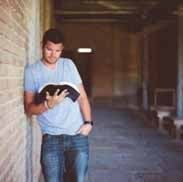# Review – Chemistry Answers EssayKenneth McQuaid
question

 A proton has a mass of ___ and a relative charge of ___

 1; +1
question

 A neutron has a relative mass of __ and a relative charge of ____.

 1;0
question

 An electron has a relative mass of __ and a relative charge of __.

 1/1836; -1
question

 The nucleus is at the center of the atom and contains the protons and neutrons. Protons and neutrons are collectively known as

 nucleons
question

 The atomic number of the atom is the number of

 protons
question

 the mass number of the atom is

 the number of protons + the number of neutrons. Also called the nucleon number.
question

 Isotopes

 atoms which have the same atomic number but different mass numbers. They have the same number of protons but different numbers of neutrons.
question

 Atoms are electrically neutral, and the positiveness of the protons is balanced by the negativeness of the electrons. It follows that in a neutral atom…

 the number of electrons equals the number of protons
question

 The electrons are found at considerable distances from the nucleus in a series of levels called energy levels. Which level holds how many electrons?

 level 1- 2 electrons level 2- 8 electrons level 3- 8 electrons. Electrons will always go into the lowest possible energy level provided there is space.
question

 Heisenberg Uncertainty Principle